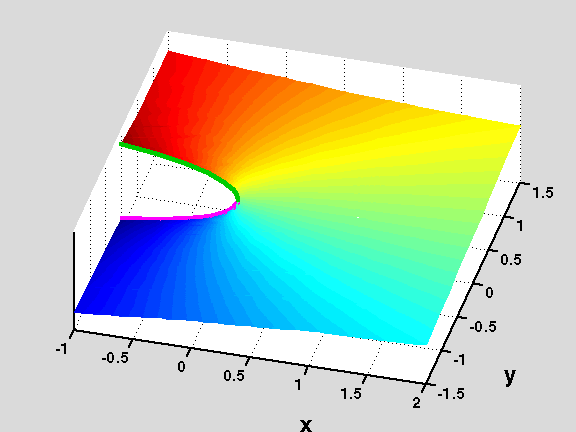18.04 | Fall 1999 | Undergraduate

# Complex Variables with Applications

Study Materials

## Riemann Surfaces: f(z)=log(1-z1/2) View 3

(All images created with MATLAB® software)

Surface generated by one of the branches where z=1 is not a branch point of f(z)=log(1-z1/2). The other branches differ from this one by a multiple of 2πi and they give rise to infinitely many copies of this surface.

These surfaces are used (in the construction of the Riemann Surface for f=f(z)) to close the gaps left when joining the surfaces given by the branches where z=1 is a branch point. The gaps are closed by matching colors (green to green and magenta to magenta) when joining the lips of the branch cut on the negative real axis. Notice that this last step must be done by going into the fourth dimension. Thus, the Riemann surface for f(z) is an object in four dimensional space.

Picture obtained using the script RiemannSur in the Athena 18.04 MATLAB® Toolkit.Figure 3: Surface generated by one of the branches where z=1 is not a branch point of f(z)=log(1-z1/2).